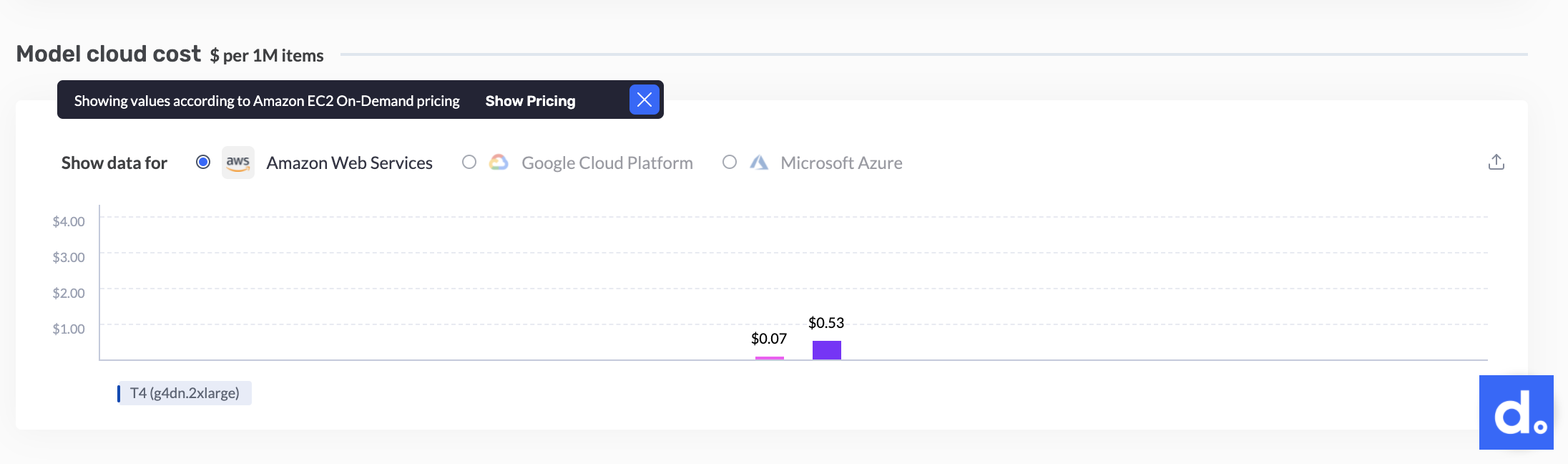# Cost Analysis Section

The Cost Analysis area shows an estimation of what it would cost to serve the model (or two models if being compared) with your cloud provider, using the \$ per 1 million frames metric.

A color-coded bar represents the cost of each model on the chosen hardware with the chosen cloud provider, as shown below (T4 machine, an AWS cloud instance g4dn.2xlarge) –One can easily see that the optimized model (pink) cost per 1 million samples is \$0.07, while the baseline (purple) cost per 1 million samples is \$0.53.

## How is the cost-to-serve being calculated?

Deci connects with the on-demand pricing of each cloud machine. We then multiply the on-demand pricing by 1 million [samples], divide by throughput [FPS] , and then manipulate the result (divide by 3600) so it will be in the same units as the on-demand pricing [\$ per hour].